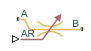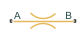# Local Restriction (TL)

Restriction in flow area in thermal liquid network

• Library:
• Simscape / Foundation Library / Thermal Liquid / Elements

•## Description

The Local Restriction (TL) block models the pressure drop due to a localized reduction in flow area, such as a valve or an orifice, in a thermal liquid network.

Ports A and B represent the restriction inlet and outlet. The input physical signal at port AR specifies the restriction area. Alternatively, you can specify a fixed restriction area as a block parameter.

The block icon changes depending on the value of the Restriction type parameter.

Restriction TypeBlock Icon

`Variable``Fixed`The restriction is adiabatic. It does not exchange heat with the environment.

The restriction consists of a contraction followed by a sudden expansion in flow area. The fluid accelerates during the contraction, causing the pressure to drop. In the expansion zone, if the Pressure recovery parameter is set to `off`, the momentum of the accelerated fluid is lost. If the Pressure recovery parameter is set to `on`, the sudden expansion recovers some of the momentum and allows the pressure to rise slightly after the restriction.

Local Restriction Schematic### Mass Balance

The mass balance in the restriction is

`$0={\stackrel{˙}{m}}_{\text{A}}+{\stackrel{˙}{m}}_{\text{B}},$`

where:

• ${\stackrel{˙}{m}}_{\text{A}}$ is the mass flow rate into the restriction through port A.

• ${\stackrel{˙}{m}}_{\text{B}}$ is the mass flow rate into the restriction through port B.

### Momentum Balance

The pressure difference between ports A and B follows from the momentum balance in the restriction:

`$\begin{array}{l}\Delta p=\frac{1}{2\rho }\left(1-\frac{{S}_{\text{R}}^{2}}{{S}^{2}}\right){v}_{R}\sqrt{{v}_{R}^{2}+{v}_{Rc}^{2}}\\ {v}_{R}=\frac{{\stackrel{˙}{m}}_{A}}{{C}_{d}\rho {S}_{R}}\\ {v}_{Rc}=\frac{{\mathrm{Re}}_{c}\mu }{{C}_{d}\rho }\sqrt{\frac{\pi }{4{S}_{R}}}\end{array}$`

where:

• Δp is the pressure differential.

• ρ is the liquid density.

• μ is the liquid dynamic viscosity.

• S is the cross-sectional area at ports A and B.

• SR is the cross-sectional area at the restriction.

• vR is fluid velocity at the restriction.

• vRc is the critical fluid velocity.

• Rec is the critical Reynolds number.

• Cd is the discharge coefficient.

If pressure recovery is off, then

`${p}_{\text{A}}-{p}_{\text{B}}=\Delta p,$`

where:

• pA is the pressure at port A.

• pB is the pressure at port B.

If pressure recovery is on, then

`${p}_{\text{A}}-{p}_{\text{B}}=\Delta p\frac{\sqrt{1-{\left(\frac{{S}_{R}}{S}\right)}^{2}\left(1-{C}_{\text{d}}^{2}\right)}-{C}_{d}\frac{{S}_{R}}{S}}{\sqrt{1-{\left(\frac{{S}_{R}}{S}\right)}^{2}\left(1-{C}_{\text{d}}^{2}\right)}+{C}_{d}\frac{{S}_{R}}{S}}.$`

### Energy Balance

The energy balance in the restriction is

`${\varphi }_{\text{A}}+{\varphi }_{\text{B}}=0,$`

where:

• ϕA is the energy flow rate into the restriction through port A.

• ϕB is the energy flow rate into the restriction through port B.

### Assumptions and Limitations

• The restriction is adiabatic. It does not exchange heat with its surroundings.

• The dynamic compressibility and thermal capacity of the liquid in the restriction are negligible.

## Ports

### Input

expand all

Input physical signal that controls the flow restriction area. The signal saturates when its value is outside the minimum and maximum restriction area limits, specified by the block parameters.

#### Dependencies

This port is visible only if you set the Restriction type parameter to `Variable`.

### Conserving

expand all

Thermal liquid conserving port associated with the inlet or outlet of the local restriction. This block has no intrinsic directionality.

Thermal liquid conserving port associated with the inlet or outlet of the local restriction. This block has no intrinsic directionality.

## Parameters

expand all

Select whether the restriction area can change during simulation:

• `Variable` — The input physical signal at port AR specifies the restriction area, which can vary during simulation. The Minimum restriction area and Maximum restriction area parameters specify the lower and upper bounds for the restriction area.

• `Fixed` — The restriction area, specified by the Restriction area block parameter value, remains constant during simulation. Port AR is hidden.

The lower bound for the restriction cross-sectional area. You can use this parameter to represent the leakage area. The input signal AR saturates at this value to prevent the restriction area from decreasing any further.

#### Dependencies

Enabled when the Restriction type parameter is set to `Variable`.

The upper bound for the restriction cross-sectional area. The input signal AR saturates at this value to prevent the restriction area from increasing any further.

#### Dependencies

Enabled when the Restriction type parameter is set to `Variable`.

Area normal to flow path at the restriction.

#### Dependencies

Enabled when the Restriction type parameter is set to `Fixed`.

Area normal to flow path at ports A and B. This area is assumed to be the same for the two ports.

The discharge coefficient is a semi-empirical parameter commonly used to characterize the flow capacity of an orifice. This parameter is defined as the ratio of the actual mass flow rate through the orifice to the ideal mass flow rate.

Specify whether to account for pressure recovery at the local restriction outlet.

The Reynolds number for the transition between laminar and turbulent regimes. The default value corresponds to a sharp-edged orifice.

## Version History

Introduced in R2013b